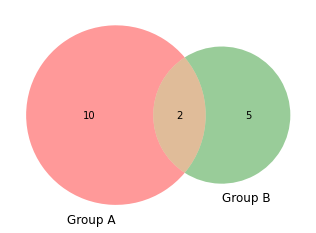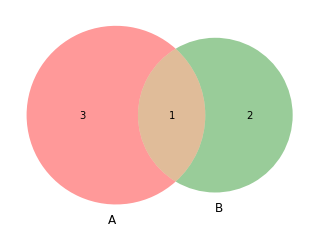# Basic Venn diagram with 2 groups

This post aims to show how to draw a basic venn diagram with an example using matplotlib library.

There are 2 main methods to make a Venn diagram with the matplotlib library, leading to the same result.

The first way is to pass directly the sizes of your groups and their intersection to the `venn2()` function. The second way is to pass 2 set of values to the function, and python will calculate itself the length of each set (=each group) and the number of common values (their intersection).

``````# library
import matplotlib.pyplot as plt
from matplotlib_venn import venn2

# First way to call the 2 group Venn diagram:
venn2(subsets = (10, 5, 2), set_labels = ('Group A', 'Group B'))
plt.show()

# Second way
venn2([set(['A', 'B', 'C', 'D']), set(['D', 'E', 'F'])])
plt.show()``````## Contact & Edit

👋 This document is a work by Yan Holtz. Any feedback is highly encouraged. You can fill an issue on Github, drop me a message onTwitter, or send an email pasting `yan.holtz.data` with `gmail.com`.